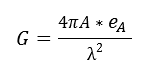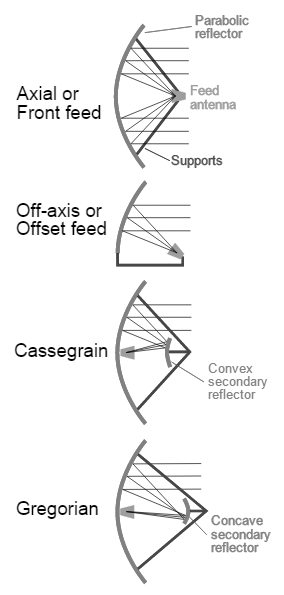# Antenna Gain Calculator

This CalcTown calculator calculates the gain of a parabolic antenna given its aperture area, aperture efficiency and wavelength of radio waves.

m2

#### ResultClick here to view image

Where,

A = aperture area of the parabolic antenna

λ = Wavelength of radio waves

eA = Efficiency of antenna aperture

G = Gain of the parabolic antenna